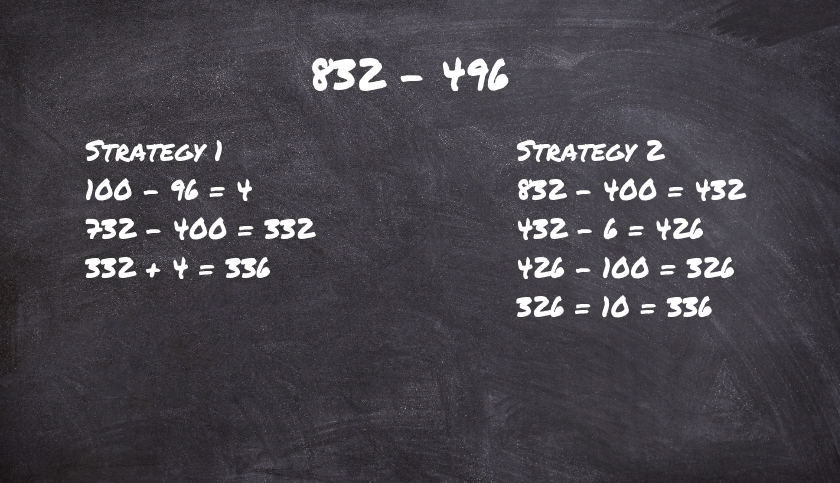# Number Talks

Two ones are two, two twos are four, two threes six, who can forget the sound of multiplication tables reverberating in their math class while growing up. These were the only words audible in a math class. Growing up, math was a set of rules and procedures to memorise by rote. Math discussion was unheard of. Precedent was given to skill and drill over deeper conceptual understanding. Whether you understood the rules or not didn’t matter – you had to know them by heart. While learning this way worked for a few, for the majority of us math was and continues to be a fearsome dragon. We are fearful of math and, when called on, unable to work out even the simplest calculations without dread.
Two ones are two, two twos are four, two threes six, who can forget the sound of multiplication tables reverberating in their math class while growing up. These were the only words audible in a math class. Growing up, math was a set of rules and procedures to memorise by rote. Math discussion was unheard of. Precedent was given to skill and drill over deeper conceptual understanding. Whether you understood the rules or not didn’t matter – you had to know them by heart. While learning this way worked for a few, for the majority of us math was and continues to be a fearsome dragon. We are fearful of math and, when called on, unable to work out even the simplest calculations without dread.
At B.D. Somani, things are a little bit different. Our Primary school students actually enjoy Mathematics. We make Mathematics interesting and real for our students. We also ensure mathematics lessons are tied into their real-world experiences. And one of the biggest reasons for our students’ joy in Mathematics is the emphasis laid on ‘Talk’ in our math class. Students are encouraged to verbalise and share their understanding of a math problem, the strategy they used to solve it, the challenges they faced and the questions they have. Math talk helps students to explain their thoughts to others, and it also provides teachers an understanding of the students’ thinking. Number Talk, Dot Talk, What Do You Notice, Which One Does Not Belong are some math activities that promote math talk. Let’s look at Number Talk, inspired by the work of Sherry Parrish and implemented effectively in all our primary classrooms and many schools world-wide.

### What are Number Talks?

Number Talks are short discussions, lasting 5 to 15 minutes. Each session focusses on student solutions for a single, carefully chosen mental math computation problem. Developed as a tool to engage students in mental math by solving interesting mathematics problems, Number Talks, practised daily, help students build number sense and develop a deeper understanding of various concepts. This gives students the ability to play with numbers, visualize problems in their minds, perform calculations in ways that makes sense to them and become flexible in their problem-solving approaches. Students are able to identify multiple solutions for each problem and also cross-check and verify each solution.

### How do Number Talks Work?

Number Talks are short discussions, lasting 5 to 15 minutes. Each session focusses on student solutions for a single, carefully chosen mental math computation problem. Developed as a tool to engage students in mental math by solving interesting mathematics problems, Number Talks, practised daily, help students build number sense and develop a deeper understanding of various concepts. This gives students the ability to play with numbers, visualize problems in their minds, perform calculations in ways that makes sense to them and become flexible in their problem-solving approaches. Students are able to identify multiple solutions for each problem and also cross-check and verify each solution.
When students are ready to answer, they use signs to communicate to the teacher that they have a solution. Signs can also be expanded to show how many solutions they have. Each student then shares their answer. The teacher writes down all solutions and then asks some students to explain the solution with all steps involved in it. Reading through the responses helps students clarify their thinking and the underlying concepts. When students get a different answer, they are taken through it with a step-by-step breakdown. This Socratic method of problem-solving ensures that students learn how to find multiple solutions to increasingly complex problems.

Let’s try another exampleMultiple strategies lead to an efficient method of solving the same problem.
Let’s try another oneNumber Talks help students absorb mathematical concepts while ensuring they develop stronger problem-solving techniques and the ability to approach any problem from multiple perspectives, helping students see the different ways that can be adopted to reach the same solution. The method used, though different, is the one that makes more sense to that student. It is her method, and she has full ownership of it. Number Talks are not limited to whole number computations, and are used to strengthen the understanding of fractions, decimals and percentages, too.
Let’s try another one
Why don’t you give it a try too? How many ways can you arrive at the number 7 using groupings in this picture?Regular practise will help your children and also you to strengthen your number sense and computation skills. You can begin by solving this problem in your mind and letting us know all your solutions in the comments. 832 – 496